Technical Article

# Clean Power for Every IC, Part 2: Choosing and Using Your Bypass Capacitors

September 27, 2015 by Robert Keim

## Proper component selection and careful PCB layout are integral to power supply bypassing.

Proper component selection and careful PCB layout are integral to power supply bypassing.

#### Capacitance: How Much Is Enough?

At the end of the previous article, we introduced the idea that a particular capacitor’s performance as part of a power-supply bypass network is dependent upon two of its nonideal characteristics, namely equivalent series resistance (ESR) and equivalent series inductance (ESL). Actually, it turns out that the part’s exact capacitance is not particularly important in the context of power-supply bypassing. This is why IC manufacturers can confidently offer the same recommendation—“0.1 µF ceramic capacitor at each power pin”—for a wide variety of analog and digital ICs. Why is the capacitance of relatively minor importance? Well, recall that capacitance is simply the ratio of charge stored on the capacitor’s plates to the voltage across the capacitor: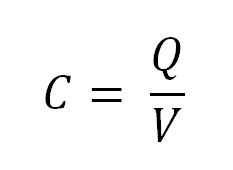So capacitance tells you how much charge the capacitor can store per volt across the capacitor. If a fully charged 10 µF and 0.1 µF capacitor are in parallel between ground and a 5 V power rail, the larger capacitor has 50 × 10-6 coulombs of charge (10 × 10-6 coulombs per volt) and the smaller one has 0.5 × 10-6 coulombs (0.1 × 10-6 coulombs per volt).

How much charge is this in relation to a power-supply bypassing application? Let’s take a look: Current (in amperes) is defined as the amount of charge (in coulombs) flowing through a conductor per unit time (seconds). Another way to express this is with a derivative: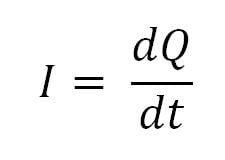Current, then, is the rate of change of charge with respect to time. This means that if we integrate current with respect to time, we have total charge: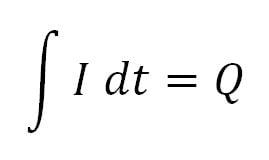Now, let’s go back to the simulated power-supply disturbances discussed in the previous article. The following current disturbance is generated in the circuit with 8 inverters and 1 nH of parasitic inductance in series with the power-supply source resistance: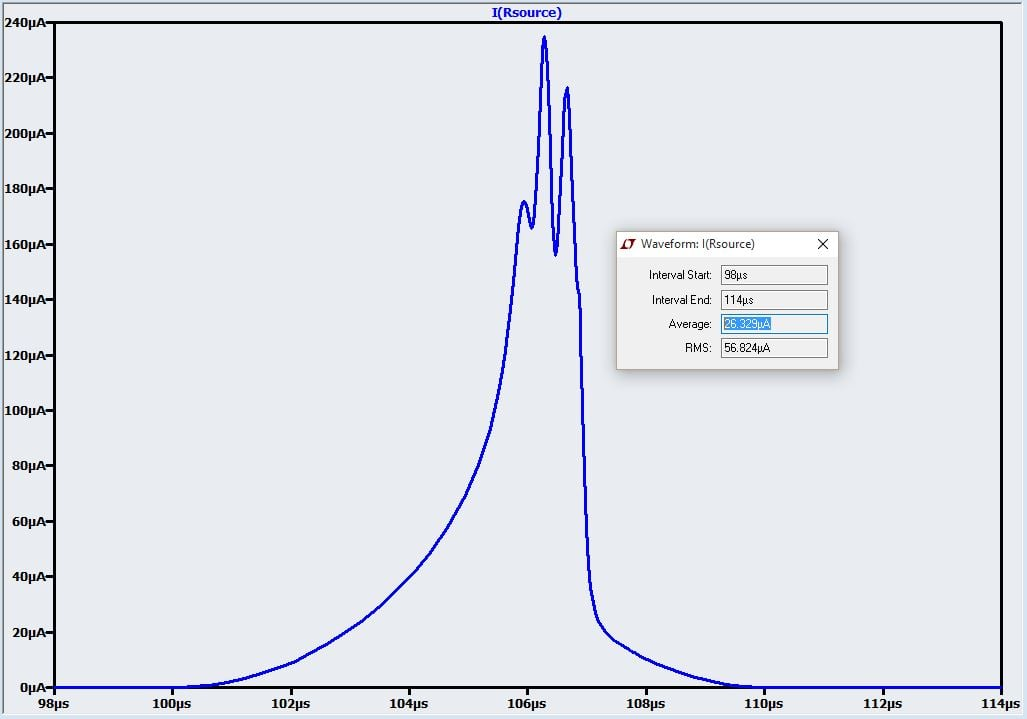LTSpice doesn’t give us the actual integration, but we can calculate it by multiplying the average current (26.3 µA) by the interval (114 µs - 98 µs = 16 µs). Thus, the total charge required to compensate for this disturbance is 26.3 µA × 16 µs = 4.2 × 10-10 coulombs. This is about 1000 times less charge than we have stored in our 0.1 µF capacitor.

This simulation is highly simplified—the amount of charge required would depend on the number of inverters in the IC, the electrical characteristics of the transistors, and so forth. Nonetheless, we can still conclude based on these calculations that a single 0.1 µF capacitor can store much more charge than is required to compensate for high-frequency current spikes generated by digital switching behavior. And this, in turn, demonstrates why the exact capacitance of a bypass capacitor is not particularly important: as long as the capacitor can store enough charge, the capacitance value is acceptable. It turns out that 0.1 µF is a convenient value, but 1 µF or even 0.01 µF might be equally appropriate in terms of capacitance.

So now we have another question to confront: Clearly a 10 µF capacitor would provide more than enough charge storage for bypassing requirements, so why bother with the 0.1 µF cap? This brings us back to our discussion of ESR and ESL.

#### The Secret Life of a Capacitor

As the following equivalent circuit demonstrates, there is a lot more going on inside a capacitor than mere capacitance:For this discussion we don’t need to worry about Rpar (which accounts for leakage current through the dielectric) or RDA and CDA (which together account for dielectric absorption). Thus, we have this simplified equivalent circuit: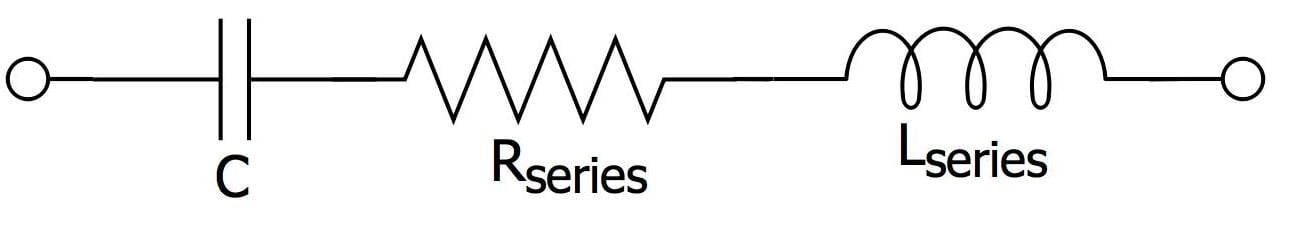The problem here should be readily apparent. Our bypass capacitor is intended to rapidly supply current during transient disturbances on the power line, yet now we have two components that impede the flow of current: a resistor, which presents a fixed impedance regardless of frequency, and an inductor, which presents higher impedance as frequency increases. At this juncture it is important to understand that ESR and ESL are determined primarily by a capacitor’s “type” (ceramic, tantalum, polymer, etc.) and package. Ceramic caps are the most popular for bypassing because they exhibit low ESR and ESL (they are also inexpensive). Next in line are tantalums; these offer moderate ESR and ESL along with high capacitance-to-volume ratio, and thus they are used for higher-value bypass capacitors intended to compensate for lower-frequency variations in the power line. For both ceramic and tantalum caps, larger packages generally correspond to higher ESL. The following table, taken from a technical report published by AVX Corporation, lists ESL values for different surface-mount packages:

Case SizeInductance (pH)
0603 (ceramic)850
0805 (ceramic)1050
1206 (ceramic)1250
1210 (ceramic)1020
0805 (tantalum)1600
1206 (tantalum)2200
1210 (tantalum)2250
2312 (tantalum)2800

Incorporating ESR considerations into the design process is fairly straightforward: small-value capacitors intended to deal with high-frequency power-line noise must have low ESR. The ESL factor, though, is somewhat more complicated. The following plot shows the impedance of a 0.1 µF, 0603 ceramic capacitor with 850 pH of ESL and 50 mΩ of ESR: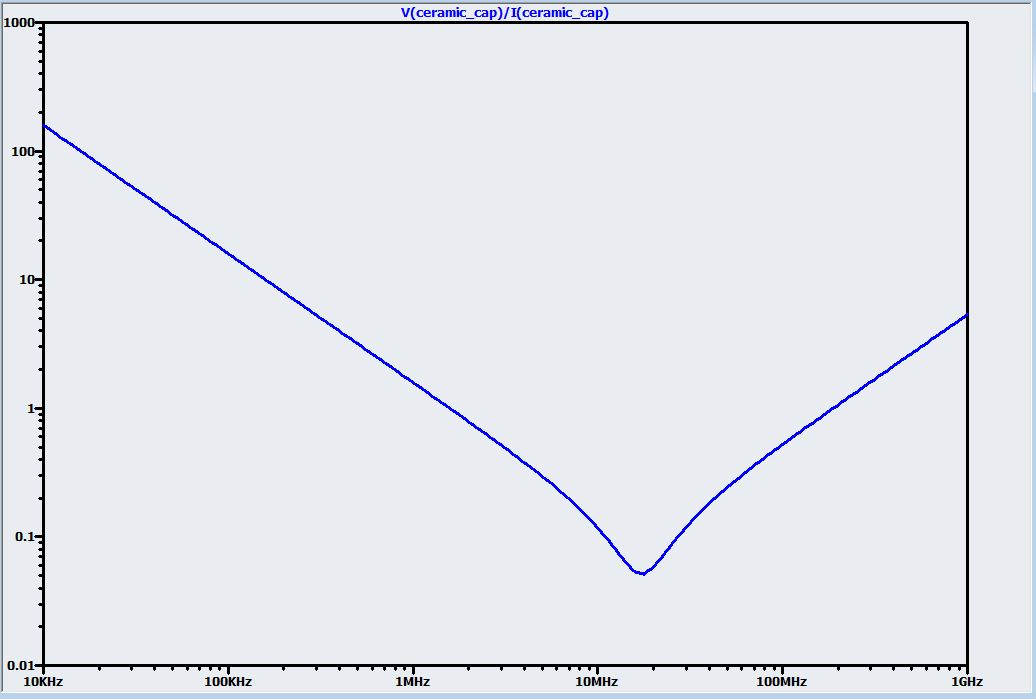As discussed in the previous article, a bypass capacitor should provide a low-impedance path that allows high-frequency noise to “pass by” the IC on its way to the circuit’s ground node. An ideal capacitor would accomplish this easily, since a capacitor’s impedance decreases with increasing frequency. But the above plot tells a different story: at a certain frequency the ESL starts to dominate the capacitance, such that the impedance actually begins to rise with frequency. Now let’s imagine that instead of the ceramic cap above we decided to use a 1 µF tantalum capacitor with 2200 pH of ESL and 1.5 Ω of ESR: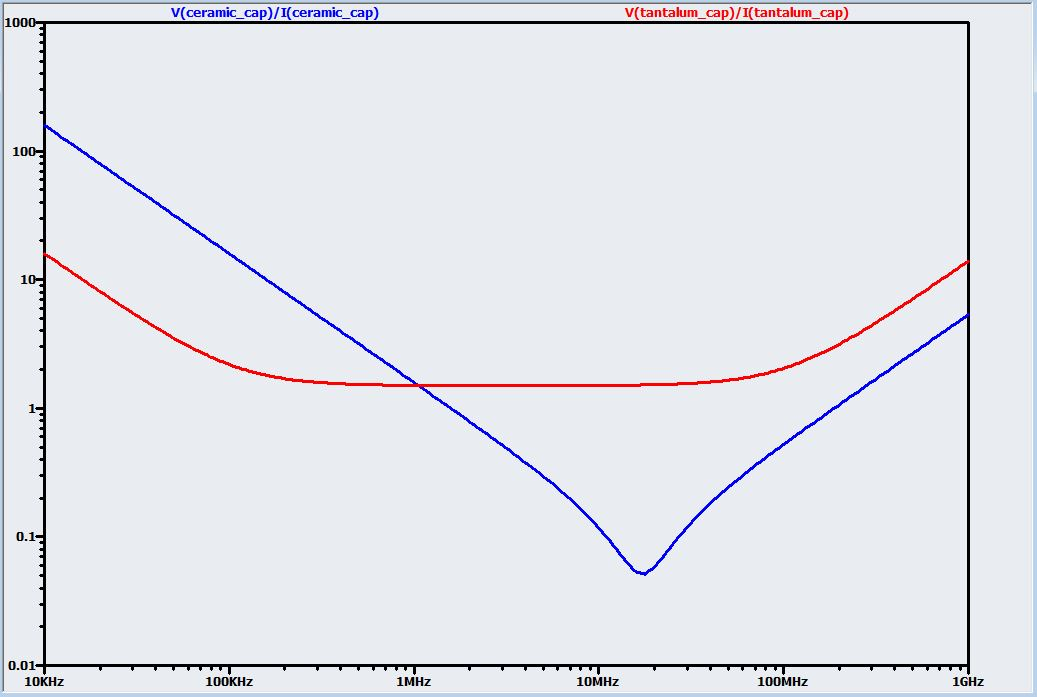The impedance of the tantalum starts out lower than that of the ceramic because of its higher capacitance, but the effect of higher ESR and ESL causes the impedance to level out at around 100 kHz, such that the impedance of the ceramic is actually 10 times lower than that of the tantalum at 10 MHz. So, if the circuit is susceptible to noise at frequencies around 10 MHz, the ceramic capacitor would be far more effective than the tantalum capacitor, even though the tantalum has higher capacitance. Furthermore, if we are dealing with very high noise frequencies, even this ceramic cap might present too much impedance. In such a case, we would need lower ESL, which means a smaller package. This plot compares the original 0603 cap with a 0.01 µF ceramic with only 500 pH of ESL (a value that might be achievable with the 0402 package):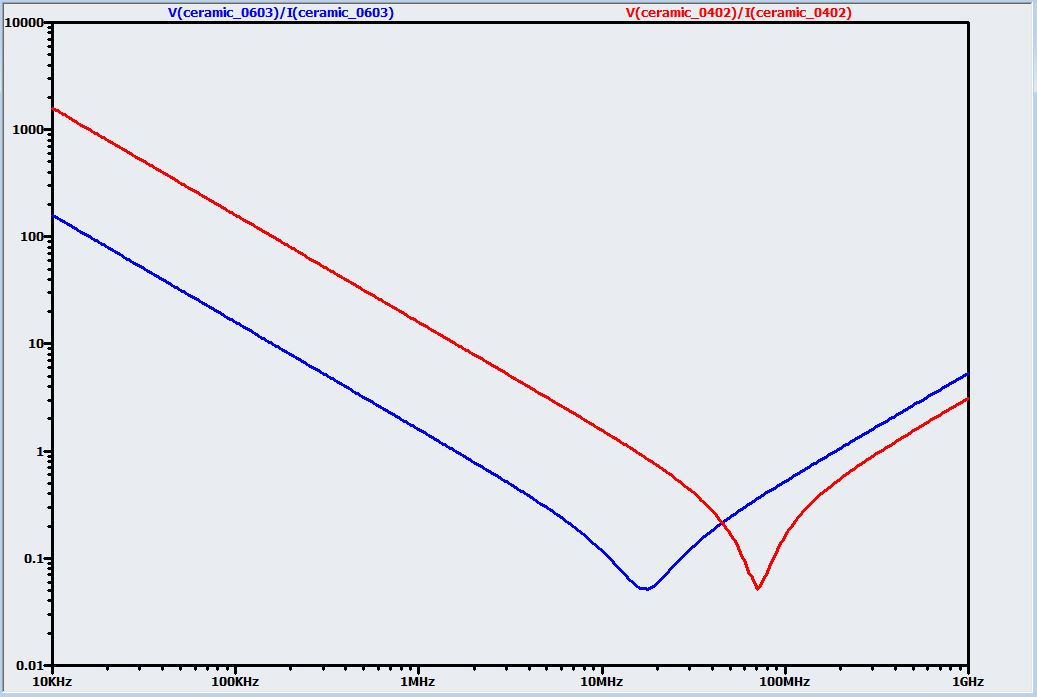At first glance it seems like we can’t win: the 0402 cap improves the high-frequency performance, but the impedance is worse than that of the 0603 from low frequency all the way to 50 MHz. We can win, though: we can put all three of these capacitors in parallel, and at any particular frequency the overall impedance will be determined by the lowest impedance among the three.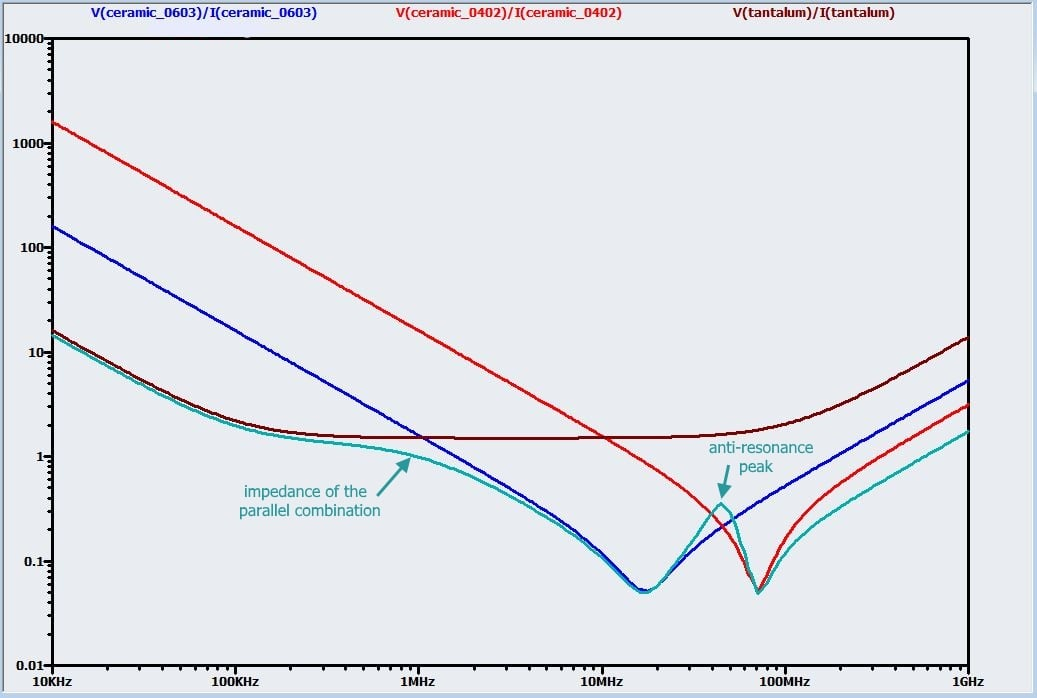So now we have a bypass network that maintains relatively low impedance over a very wide range of frequencies. The only surprise here is the peak at 50 MHz where the overall impedance is higher than the individual impedances. This is referred to as an anti-resonance peak, and you need to watch out for these wherever a decreasing (i.e., capacitance-dominant) impedance intersects with an increasing (i.e., inductance-dominant) impedance.

#### Don’t Ruin a Good Design with a Bad Layout

Proper PCB layout is a critical aspect of bypass design—for example, engineers at Texas Instruments found that extending the distance between a 0.1 µF cap and the IC’s power pin from 0.3 inches to 1 inch increased the power-line ringing amplitude from 250 mV to 600 mV. Fortunately, the rules for laying out bypass capacitors are simple: minimize resistance, minimize inductance. This is accomplished by placing the capacitor as close to the power pin as possible and using the shortest possible traces for all connections. Ideally, both the ground and the power rail can be accessed through vias to planes: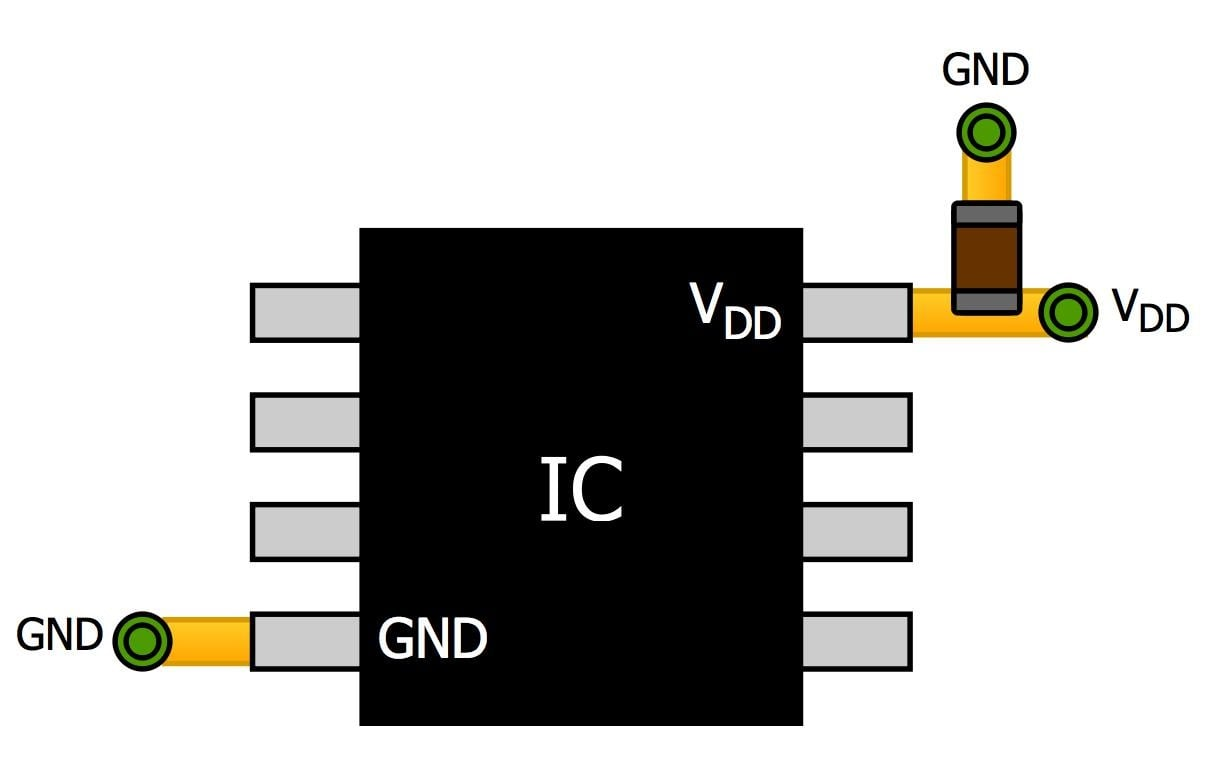#### Bypass Cap Recap

We now have enough information to formulate a concise set of guidelines for successful bypassing:

• When in doubt, give each power pin a 0.1 µF ceramic cap, preferably size 0805 or smaller, in parallel with a 10 µF tantalum or ceramic.
• You can probably omit the 10 µF cap, or replace it with something smaller, if you are concerned only about high-frequency noise.
• If you need to compensate for long-term supply deviations that will require large amounts of stored charge, you may need to give each IC an additional larger capacitor, say 47 µF.
• If your design includes very high frequencies or particularly sensitive circuitry, use a simulator to analyze the AC response of your bypass network. (It might be difficult to find solid specs for ESR and ESL, especially considering that capacitor ESR can vary significantly with frequency—just do the best you can.) If necessary, include lower-ESL ceramic caps to improve the high-frequency impedance characteristics.
• Locate the high-frequency ceramic caps as close to the power pin as possible, and use short traces and vias to minimize parasitic inductance and resistance. The location of larger capacitors intended for low-frequency bypassing is not quite as critical, but these also should be close to the IC—within a half-inch or so.

Next Article in Series: Clean Power for Every IC, Part 3: Understanding Ferrite Beads•roderick young September 30, 2015

Great article, thanks!  Another choice for capacitor position would be on the other side of the board, directly under the IC, if that’s an option.

Like.
•salihyanikgonul October 07, 2016

Excellent explanation and good foundation of solid facts!

Like.
•Gökhan Çetinkaya June 03, 2018

it is perfect information. thank you for all.

Like.